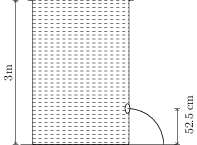# Bernoulli's Theorem and its Applications

## Problems from IIT JEE

Problem (IIT JEE 2005):Water is filled in a beaker upto a height of 3 m. An orifice (hole) is at a height of 52.5 cm from the bottom of beaker (see figure). The ratio of cross-sectional area of the orifice and the beaker is $0.1$. The square of the speed of the liquid coming out from the orifice is, (Take $g={10}\;\mathrm{m/s^2}$.)

1. ${50}\;\mathrm{(m/s)^2}$
2. ${50.5}\;\mathrm{(m/s)^2}$
3. ${51}\;\mathrm{(m/s)^2}$
4. ${52}\;\mathrm{(m/s)^2}$

Solution: Let $A_1$ be the cross-sectional area of the beaker and $v_1$ be the flow velocity at a height $h_1={3}\;\mathrm{m}$. Let $A_2$ be the cross-sectional area of the orifice and $v_2$ be the flow velocity at the orifice located at a height $h_2={0.525}\;\mathrm{m}$. Using continuity equation on an imaginary streamline from top of the beaker to the orifice, $A_1v_1=A_2v_2$, we get, \begin{align} \label{lkb:eqn:1} v_1=(A_2/A_1)v_2. \end{align} Bernoulli's equation, $P_0+\rho g h_1+\frac{1}{2}\rho v_1^2=P_0+\rho g h_2+\frac{1}{2}\rho v_2^2$, gives, \begin{align} \label{lkb:eqn:2} v_2^2=v_1^2+2g(h_1-h_2). \end{align} Eliminate $v_1$ from above equations to get, \begin{align} v_2^2=\frac{2g(h_1-h_2)}{1-({A_2}/{A_1})^2}=\frac{2(10)(3-0.525)}{1-0.01}={50}\;\mathrm{m^2/s^2}.\nonumber \end{align}